﻿ 基于BP神经网络和拟合对收得率的预测

# 基于BP神经网络和拟合对收得率的预测Prediction of Yield Based on BP Neural Network and Fitting

Abstract: Deoxidation alloying in the steelmaking process is an important process link in steel smelting. In this paper, a prediction model for the carbon and manganese yield is studied and established, and the simulation optimization test is defined. The control space theorem is defined to judge the model prediction accuracy. Secondly, using BP neural network and fitting methods, the prediction models of alloy element yield in the process of deoxidizing alloying are established respectively, which shortens the learning and training time and improves the prediction accuracy of the model. The predicted values of BP neural network are both 85%. Above, the fitting prediction values are all above 82%. The results show that: 100 production times are randomly selected for simulation optimization. According to the control interval theorem, the fitting prediction accuracy rate is above 84%, and the BP neural network prediction accuracy rate is above 89%, and the BP neural network prediction model is more in line with production requirements.

1. 引言

2. 收得率预测评判标准

$合金元素\text{ }\text{ }i\text{ }\text{ }量=\frac{目标出钢含量\text{ }\text{ }i-吹止成分\text{ }\text{ }i}{元素\text{ }\text{ }i\text{ }\text{ }收得率}×\left(钢水净重-合金总质量\right)$

2.1. BP神经网络的C、Mn收得率预测模型建立

BP神经网络是一种非线性动力系统，包含输入层、隐含层、输出层三层单元。设输入向量为 $X={\left({x}_{1},{x}_{2},\cdots ,{x}_{n}\right)}^{\text{T}}$，隐含层输出向量为 $Y={\left({y}_{1},{y}_{2},\cdots ,{y}_{m}\right)}^{\text{T}}$，输出层输出向量为 $G={\left({g}_{1},{g}_{2},\cdots ,{g}_{k}\right)}^{\text{T}}$，则有期望输出向量为 $D={\left({d}_{1},{d}_{2},\cdots ,{d}_{l}\right)}^{\text{T}}$，输出层到隐含层之间的权值矩阵用V表示， $V=\left({v}_{1},{v}_{2},\cdots ,{v}_{m}\right)$，其中列向量 ${v}_{m}$ 为隐含层第m个神经元对应的权向量，隐含层到输出层之间的权值矩阵用W表示， $W=\left({w}_{1},{w}_{2},\cdots ,{w}_{i}\right)$，其中列向量 ${w}_{i}$ 为输出层第i个神经元对应的权向量 。

$\left\{\begin{array}{l}{G}_{j}=f\left(ne{t}_{j}\right),j=1,2,\cdots ,k,\text{\hspace{0.17em}}输入层\\ ne{t}_{j}=\underset{i=0}{\overset{m}{\sum }}{\omega }_{ij}{g}_{i},j=1,2,\cdots ,k,\text{\hspace{0.17em}}隐含层\\ f\left(x\right)=\frac{1}{1+{\text{e}}^{-x}},\text{\hspace{0.17em}}转移函数\\ {f}^{\prime }\left(x\right)=f\left(x\right)\left[1-f\left(x\right)\right]\end{array}$

$E=\frac{1}{2}{\left(D-G\right)}^{2}=\frac{1}{2}\underset{i=1}{\overset{k}{\sum }}{\left({d}_{i}-{g}_{i}\right)}^{2}$ (1)

$\begin{array}{c}E=\frac{1}{2}\underset{i=1}{\overset{k}{\sum }}{\left[{d}_{i}-f\left(ne{t}_{i}\right)\right]}^{2}\\ =\frac{1}{2}\underset{i=1}{\overset{k}{\sum }}{\left[{d}_{i}-f\left(ne{t}_{i}=\underset{i=0}{\overset{m}{\sum }}{w}_{ij}{g}_{i}\right)\right]}^{2}\end{array}$ (2)

$\begin{array}{c}E=\frac{1}{2}\underset{i=1}{\overset{k}{\sum }}{\left\{{d}_{i}-f\left[\underset{i=0}{\overset{m}{\sum }}{w}_{ij}f\left(ne{t}_{i}\right)\right]\right\}}^{2}\\ =\frac{1}{2}\underset{i=1}{\overset{k}{\sum }}{\left\{{d}_{i}-f\left[\underset{i=0}{\overset{m}{\sum }}{w}_{ij}f\left(\underset{i=0}{\overset{n}{\sum }}{v}_{ij}{g}_{i}\right)\right]\right\}}^{2}\end{array}$ (3)

$\begin{array}{l}\Delta {w}_{ij}=-\eta \frac{\partial E}{\partial {w}_{ij}},\text{}i=0,1,2,\cdots ,n;\text{}j=1,2,\cdots ,k\\ \Delta {v}_{mi}=-\eta \frac{\partial E}{\partial {v}_{mi}},\text{}m=0,1,2,\cdots ,l;\text{}i=1,2,\cdots ,n\end{array}$ (4)

2.2. BP神经网络的C、Mn收得率预测模型求解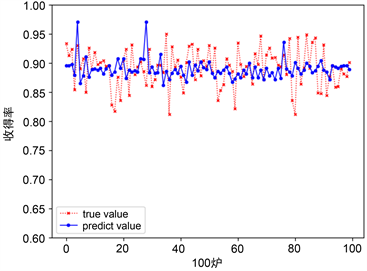Figure 1. Comparison of real value and predicted value of Mn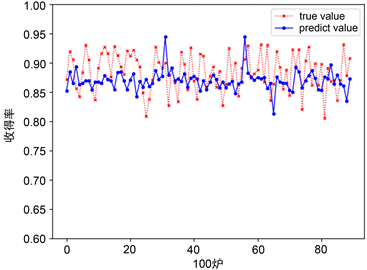Figure 2. Comparison of real value and predicted value of C

2.3. 拟合的C、Mn收得率预测模型求解Table 1. 10 group year (year) and yield (%)

$y={a}_{1}+{a}_{2}x+\cdots +{a}_{n}{x}^{n-1}$ (5)

$\underset{i}{\overset{n}{\sum }}{\delta }_{i}^{2}=\mathrm{min}$

$\left\{{r}_{1}\left(x\right),{r}_{2}\left(x\right),\cdots ,{r}_{m}\left(x\right)\right\}=\left\{1,x,{x}^{2},\cdots ,{x}^{m-1}\right\}$ (6)

$\phi \left(x\right)={a}_{1}{r}_{1}\left(x\right)+{a}_{2}{r}_{2}\left(x\right)+\cdots +{a}_{m}{r}_{m}\left(x\right)$

$J\left({a}_{1},{a}_{2},\cdots ,{a}_{m}\right)=\underset{i}{\overset{n}{\sum }}{\delta }_{i}^{2}=\underset{i}{\overset{n}{\sum }}{\left[\phi \left({x}_{i}\right)-{y}_{i}\right]}^{2}=\underset{i}{\overset{n}{\sum }}{\left[\underset{k=1}{\overset{m}{\sum }}{a}_{k}{r}_{k}\left({x}_{i}\right)-{y}_{i}\right]}^{2}$ (7)

$\frac{\partial J}{\partial {a}_{k}}=0\text{}\left(k=1,\cdots ,m\right)$ (8)

$\left(\begin{array}{cccc}\left({r}_{1},{r}_{1}\right)& \left({r}_{1},{r}_{2}\right)& \cdots & \left({r}_{1},{r}_{m}\right)\\ \left({r}_{2},{r}_{1}\right)& \left({r}_{2},{r}_{2}\right)& \cdots & \left({r}_{2},{r}_{m}\right)\\ ⋮& ⋮& \ddots & ⋮\\ \left({r}_{m},{r}_{1}\right)& \left({r}_{m},{r}_{2}\right)& \cdots & \left({r}_{m},{r}_{m}\right)\end{array}\right)\left(\begin{array}{c}{a}_{1}\\ {a}_{2}\\ ⋮\\ {a}_{m}\end{array}\right)=\left(\begin{array}{c}\left(y,{r}_{1}\right)\\ \left(y,{r}_{2}\right)\\ ⋮\\ \left(y,{r}_{m}\right)\end{array}\right)$

$Q=\left[\begin{array}{ccc}{r}_{1}\left({x}_{1}\right)& \cdots & {r}_{m}\left({x}_{1}\right)\\ ⋮& \ddots & ⋮\\ {r}_{1}\left({x}_{n}\right)& \cdots & {r}_{m}\left({x}_{n}\right)\end{array}\right]$$a=\left[\begin{array}{c}{a}_{1}\\ ⋮\\ {a}_{2}\end{array}\right]$$y=\left[\begin{array}{c}{y}_{1}\\ ⋮\\ {y}_{n}\end{array}\right]$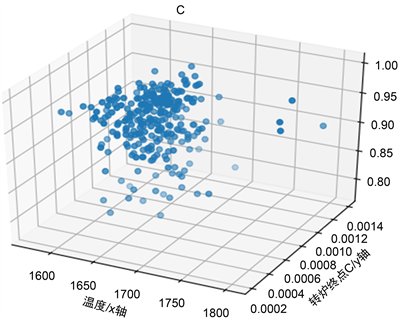Figure 3. Schematic diagram of the three-dimensional relationship between two impact factors and C yieldTable 2. C element fitting predicted value and true value analysis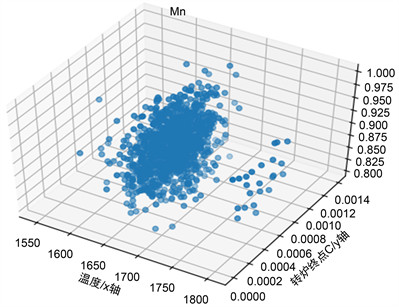Figure 4. Schematic diagram of a three-dimensional relationship between two factors and Mn yieldTable 3. Mn element fitting prediction value and true value analysis

3. 仿真优化检验

$合金元素\text{ }\text{ }i\text{ }\text{ }量=\frac{目标出钢含量\text{ }\text{ }i-吹止成分\text{ }\text{ }i}{元素\text{ }\text{ }i\text{ }\text{ }收得率}×\left(钢水净重-合金总质量\right)$Table 4. Fitting the model to predict the carbon content of the alloyTable 5. Fitting the model to predict the manganese content of the alloy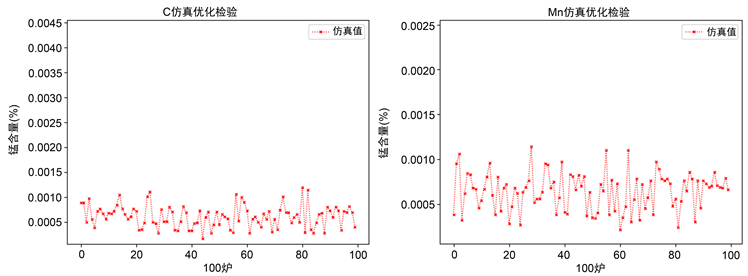Figure 5. C, Mn simulation test chart

4. 结论

 马海志. BP神经网络的改进研究及应用[D]: [硕士学位论文]. 哈尔滨: 东北农业大学, 2011.

 包燕平, 张超杰, 王敏. 炼钢过程中合金减量化研究现状及展望[J]. 工程科学学报, 2018, 40(9): 1017-1026.

 徐端, 刘士新. 数据驱动和机理模型混合的炼钢–连铸能耗建模研究[J]. 河北冶金, 2018(10): 14-19+40.

 潘军, 邓南阳, 胡惠华, 赵斌, 薛顺, 王义平. 降低低合金钢Q345B合金消耗的工艺优化[J]. 钢铁研究, 2015, 43(2): 56-58.

 Biao, T., et al. (2017) The Research Process on Converter Steelmaking Process by Using Limestone. IOP Conference Series: Earth and Environmental Science, 81, Article ID: 012175.

 韩敏, 徐俏, 赵耀, 林东, 杨溪林. 基于收得率预测模型的转炉炼钢合金加入量计算[J]. 炼钢, 2010, 26(1): 44-47.

 薛正良, 吴丽嘉, 王炜, 左都伟, 罗斌, 彭灿峰, 郝飞翔, 严明. 转炉终点钢水残锰含量及锰收得率的影响因素分析[J]. 炼钢, 2011, 27(6): 40-43.

 王广. 人工神经网络发展现状综述[J]. 中小企业管理与科技(下旬刊), 2017(2): 165-167.

Top